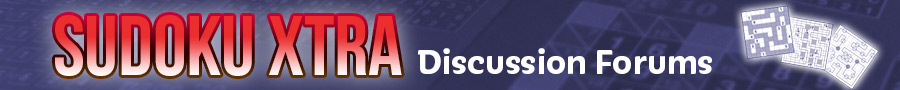Back to main Sudoku Xtra site

# So many numbers on board already, BUT STUCK

Posted by Eman
 Eman So many numbers on board already, BUT STUCK March 07, 2011 07:26AM
How would I know where a next number would go from here?
I have even put the convenience of noting the possible numbers in the blanks.
Would really appreciate anyone's help.
 Naughty Re: So many numbers on board already, BUT STUCK March 12, 2011 02:49PM
Hey Eman, I took a look at your puzzle and I have to say I can't see a way to progress from here either. However, I did input it into a Sudoku Solver I have on my DS and it did confirm there is only one valid solution.
At this stage it's usually obvious that logic alone is not sufficient to solve the puzzle. I actually hate sudokus/puzzles like this as I prefer pure logic approaches, however, the technique I often use (if I haven't already binned the uncompleted puzzle in disgust!) is to pick an isolated "either/or" pair (the 5 and 8 in the bottom-right quadrant in your example would be an ideal choice) and then mentally (or on another sheet or paper) insert both numbers arbitrarily and then progress the puzzle from there. It will usually become clear fairly quickly that the order of the two I chose was either incorrect (in which case the swapped order is the correct solution) or was the correct guess and the puzzle is one step nearer to completion (or totally complete!).
Like I said though, I'm not keen on any puzzles that rely on this mechanism for more than one step ahead...
:nathaN
 Re: So many numbers on board already, BUT STUCK March 14, 2011 04:52PM Registered: 11 years ago Posts: 2
Eman and Naughty,

Just like you, I also prefer pure logic approaches. Please have a look at the method I use to solve the puzzle mentioned by Eman . Do you accept this as a logic approach? As I don't see this method can fit in any named category available, I call it YZ (stands for YELLOW ZONE).

The method is as follows:

First round
YZ method on The Original Puzzle by Eman
Set a Yellow Zone : r3c4=13 , r5c4=36 [If r3c4<>1 , r5c4<>6 , we get a contradiction]
Find a step not allowed : r7c6=5
The logic : r7c6=5=>r8c5=3=>r5c5=6=>r5c4<>6；
r7c6=5=>r7c6<>1=>r3c6=1=>r3c4<>1。
The result : r7c6<>5

Second round
YZ method on The Original Puzzle with 5 eliminated in r7c6.
Set a Yellow Zone : r3c6=13 , r7c6=18 [If r3c6<>3 , r7c6<>8 , we get a contradiction]
Find a step not allowed : r2c5=6
The logic : r2c5=6=>r2c6=8=>=>r7c6<>8；
r2c5=6=>r5c5=3=>r5c4<>3=>r3c4=3=>r3c6<>3。
The result : r2c5<>6

We can then solve the puzzle by singles.

A brief introduction of the YZ method:
We all know 'naked pair'. If we have, say, r2c4=56 and r2c7=56, a naked pair, then r2c1=5 is not allowed . Because if r2c1=5 we get r2c4=6 and r2c7=6 hence forming a contradiction! Now if we set a YELLOW ZONE r3c4=13 , r5c4=36 in THIS PUZZLE and find a step r7c6=5 leading to r3c4=3 , r5c4=3, a contradiction . So we call this step r7c6=5 'not allowed'. In other words, we should have r7c6<>5 .

Of course, YELLOW ZONES can be chosen in various forms, you will see different forms of YELLOW ZONES in doing a puzzle given by Keith in Dailysudoku’s Forum who claimed that this is one of the puzzles that can only be solved by using brute force (guessing). I am going to post on Dailysudoku's Forum the solution using YZ method in the near future.
 Re: So many numbers on board already, BUT STUCK March 26, 2011 05:28PM Registered: 11 years ago Posts: 2
I have just told by someone that I used the YZ method two times to get the solution is not necessary . He suggested the following setting with a bit bigger Yellow Zone to solve the puzzle.
YZ method on The Original Puzzle by Eman :

```+----------+-----------------+--------------+
| 3   1  8 | 59     259  25  | 4    7   6  |
| 5   4  9 | 678    67   68  | 2    3   1  |
| 6   7  2 | 13      4   13  | 8    9   5  |
+----------+------------------+--------------+
| 8   3  6 | -15    15Y   9   | 7    2   4  |
| 9   2  7 | 36     36Y   4   | 5    1   8  |
| 1   5  4 | 2       8    7   | 3    6   9  |
+----------+------------------+--------------+
| 4   6  3 | 1578   157  158  | 9   58   2 |
| 2   9  1 | 4      35Y  358  | 6   58   7 |
| 7   8  5 | 69    269    26  | 1    4   3 |
+-----------+-----------------+--------------+```

Set a Yellow Zone : r4c5=15 , r5c5=36,r8c5=35 [Marked with Y]
[If r4c5<>1 , r5c5<>6 , we get a contradiction]
A step not allowed : r4c4=1
The logic : r4c4=1=>r4c5<>1;
r4c4=1=>r3c4=3=>r5c4=6=>r5c5<>6。
Thus we have that r4c4=1=>r458c5=35(a failure mode)
The result : r4c4=5 and then get the solution by singles.
Sorry, only registered users may post in this forum.